Question

Point O is on line segment NP‾\overline{NP}NP. Given OP=10,OP=10,OP=10, NP=2x,NP=2x,NP=2x, and NO=x+7,NO=x+7,NO=x+7, determine the numerical length of NO‾.\overline{NO}.NO.

1.quangkhai

Given:

Point O is on line segment NP.

OP=10, NP=2x, and NO=x+7.

To find:

The numerical length of NO.

Solution:

If point O is on line segment NP, then by segment addition property, we get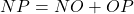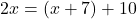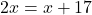Subtract both sides by x.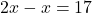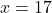The value of x is 17.

Now,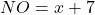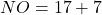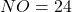Therefore, the numerical length of NO is 24units.